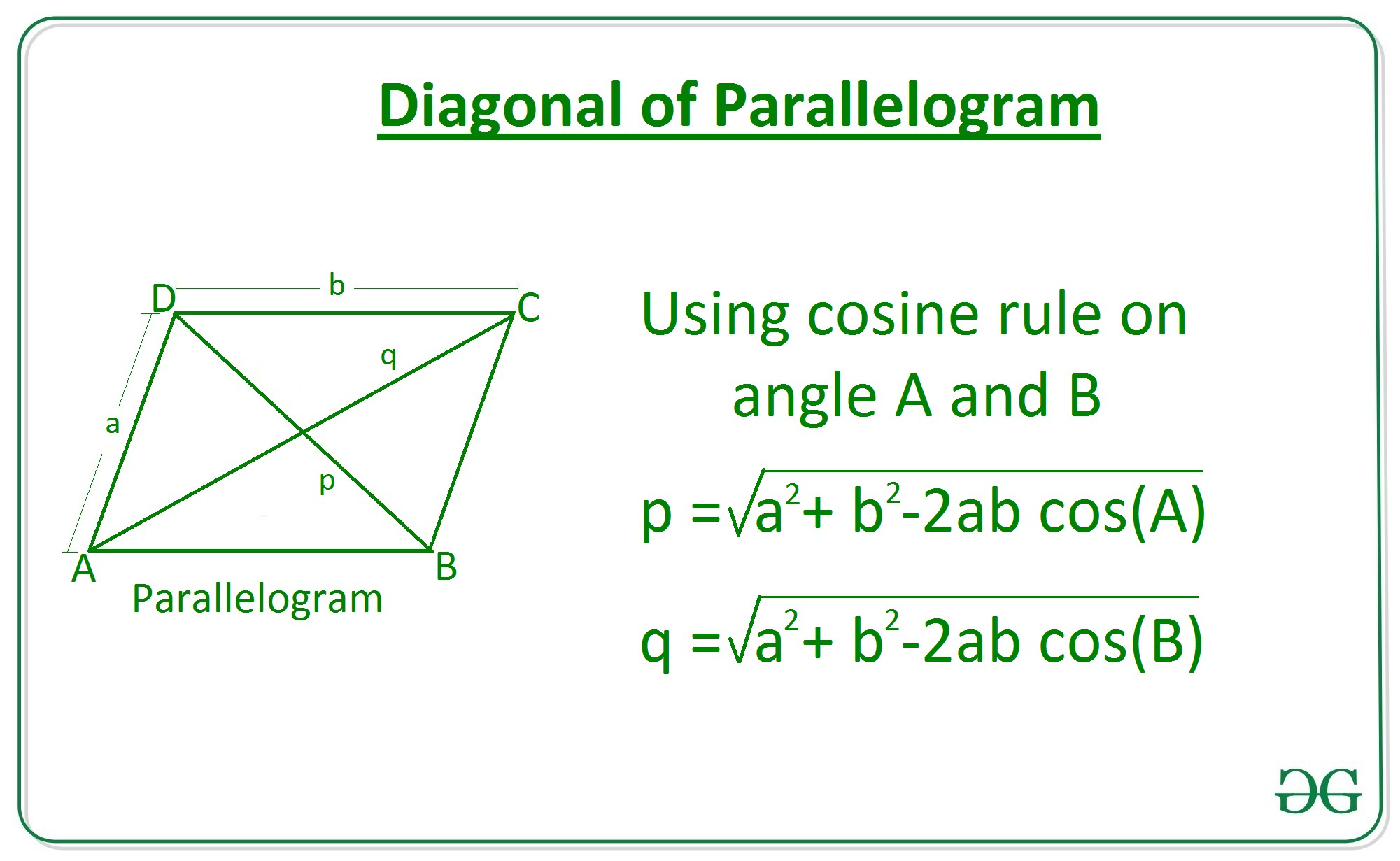# Length of diagonal of a parallelogram using adjacent sides and angle between them

• Last Updated : 08 Apr, 2021

Given two integers a and b where a and b represents the length of adjacent sides of a parallelogram and an angle 0 between them, the task is to find the length of diagonal of the parallelogram.

Examples:

Input: a = 6, b = 10, 0=30
Output: 6.14

Input: a = 3, b = 5, 0=45
Output: 3.58

Approach: Consider a parallelogram ABCD with sides a and b, now apply cosine rule at angle A in the triangle ABD to find the length of diagonal p, similarly find diagonal q from triangle ABC.Therefore the diagonals is given by:## C++

 // C++ program to find length // Of diagonal of a parallelogram // Using sides and angle between them. #include  using namespace std; #define PI 3.147   // Function to return the length // Of diagonal of a parallelogram // using sides and angle between them. double Length_Diagonal(int a, int b, double theta) {     double diagonal = sqrt((pow(a, 2) + pow(b, 2)) -                        2 * a * b * cos(theta * (PI / 180)));       return diagonal; }   // Driver Code int main() {       // Given sides     int a = 3;     int b = 5;       // Given angle     double theta = 45;       // Function call     double ans = Length_Diagonal(a, b, theta);       // Print the final answer     printf("%.2f", ans); }   // This code is contributed by Amit Katiyar

## Java

 // Java program to find length  // Of diagonal of a parallelogram  // Using sides and angle between them. class GFG{   // Function to return the length // Of diagonal of a parallelogram // using sides and angle between them. static double Length_Diagonal(int a, int b,                               double theta) {     double diagonal = Math.sqrt((Math.pow(a, 2) +                                   Math.pow(b, 2)) -                                   2 * a * b *                                   Math.cos(theta *                                   (Math.PI / 180)));       return diagonal; }   // Driver Code public static void main(String[] args) {           // Given sides     int a = 3;     int b = 5;       // Given angle     double theta = 45;       // Function call     double ans = Length_Diagonal(a, b, theta);       // Print the final answer     System.out.printf("%.2f", ans); } }   // This code is contributed by amal kumar choubey

## Python3

 # Python3 Program to find length  # Of diagonal of a parallelogram  # Using sides and angle between them.   import math       # Function to return the length # Of diagonal of a parallelogram  # using sides and angle between them.   def Length_Diagonal(a, b, theta):           diagonal = math.sqrt( ((a**2) + (b**2))     - 2 * a*b * math.cos(math.radians(theta)))           return diagonal       # Driver Code   # Given Sides a = 3 b = 5   # Given Angle theta = 45     # Function Call   ans = Length_Diagonal(a, b, theta)       # Print the final answer print(round(ans, 2))

## C#

 // C# program to find length  // Of diagonal of a parallelogram  // Using sides and angle between them. using System;   class GFG{   // Function to return the length // Of diagonal of a parallelogram // using sides and angle between them. static double Length_Diagonal(int a, int b,                               double theta) {     double diagonal = Math.Sqrt((Math.Pow(a, 2) +                                   Math.Pow(b, 2)) -                                   2 * a * b *                                   Math.Cos(theta *                                  (Math.PI / 180)));       return diagonal; }   // Driver Code public static void Main(String[] args) {           // Given sides     int a = 3;     int b = 5;       // Given angle     double theta = 45;       // Function call     double ans = Length_Diagonal(a, b, theta);       // Print the readonly answer     Console.Write("{0:F2}", ans); } }   // This code is contributed by amal kumar choubey

## Javascript

 

Output:

3.58

Time Complexity: O(1)
Auxiliary Space: O(1)

My Personal Notes arrow_drop_up
Recommended Articles
Page :